# GMAT Math : Understanding exponents

## Example Questions

1 2 3 4 5 6 7 9 Next →

### Example Question #81 : Exponentsis the additive inverse of the multiplicative inverse ofis the additive inverse of the multiplicative inverse of. Which of the following is equal to the expression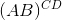regardless of the values of the variables?is an undefined quantityExplanation:

The additive inverse of a number is the number which, when added to that number, yields sum 0; the multiplicative inverse of a number is the number which, when multiplied by that number, yields product 1.

Letbe the multiplicative inverse of. Then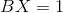, or, equivalently,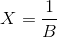.is the additive inverse of this number, so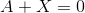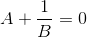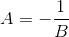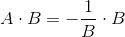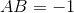By similar reasoning,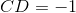, and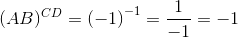### Example Question #1186 : Problem Solving Questions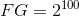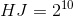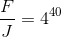Which of the following is equal to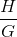?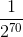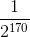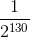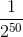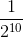Explanation:

Divide: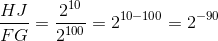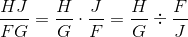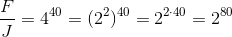Substitute: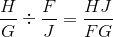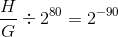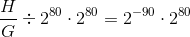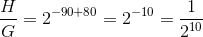### Example Question #81 : Understanding Exponentsis the additive inverse ofis the multiplicative inverse of. Which of the following is equal to the expression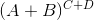regardless of the values of the variables?must be an undefined quantity.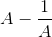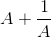Explanation:

The additive inverse of a number is the number which, when added to that number, yields sum 0. Sinceis the additive inverse of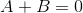The multiplicative inverse of a number is the number which, when multiplied by that number, yields product 1. Sinceis the multiplicative inverse of, then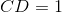, or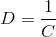.

It follows that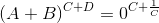.

0 raised to any nonzero power is equal to 0, and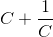must be nonzero, so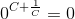, the correct response.

### Example Question #82 : Understanding Exponentsis the additive inverse ofis the additive inverse of. Which of the following is equal to the expressionregardless of the values of the variables?must be an undefined quantitymust be an undefined quantity

Explanation:

The additive inverse of a number is the number which, when added to that number, yields sum 0. Sinceis the additive inverse ofandis the additive inverse of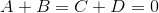and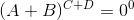,

which is an undefined expression.

1 2 3 4 5 6 7 9 Next →

### All GMAT Math Resources